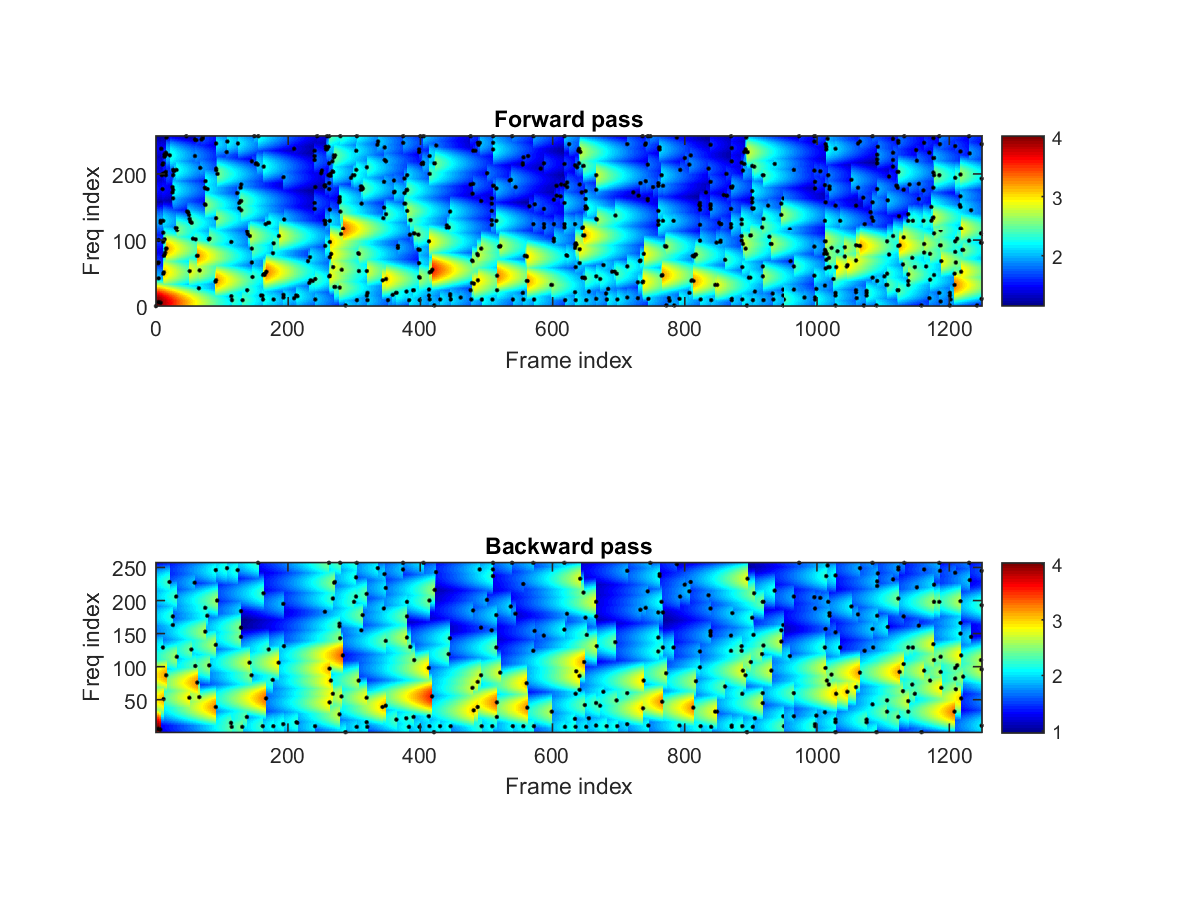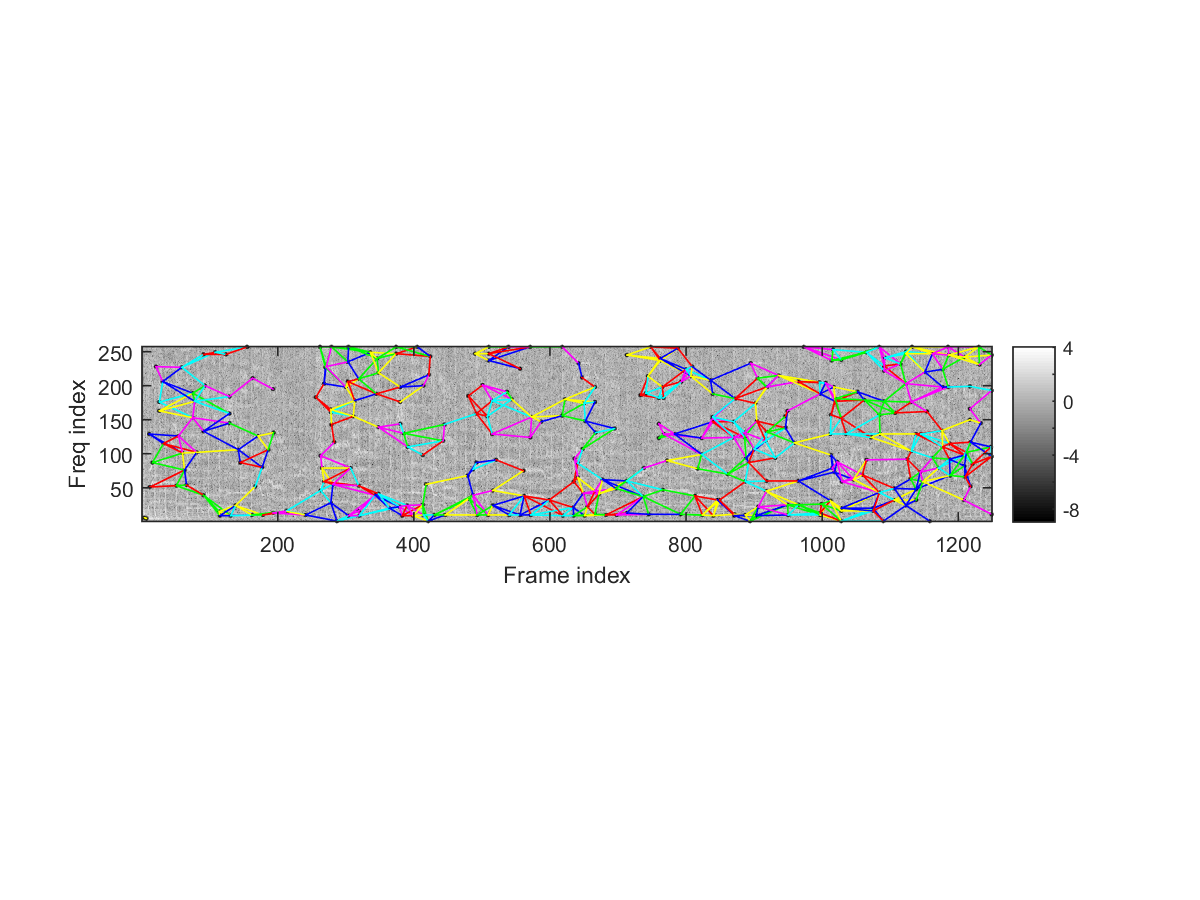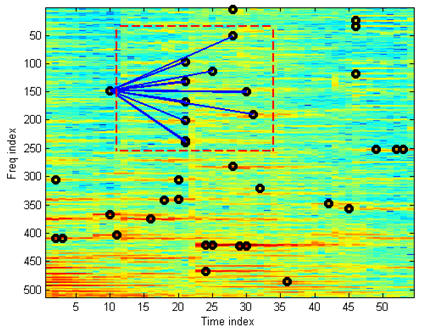## 16-1 Landmark Extraction

The goal of audio fingerprinting is to identify the source of a given noisy music recording. The query music clip must come from the original music clip, with possible channel distortion and environmental noises. Audio fingerprinting has been successfully applied to audio-based search, with successful commercial products such as Shazam, Souundhound, Intonow, and so now. This section will describe one of the most adopted algorithm for audio fingerprinting.

One of the robust features that can resist channel distortion and environmental noise is salient peaks in a spectrogram. Salient peaks in a spectrogram can be defined as the local maxima along time and frequency. In practice, since there are too many local maxima, we usually need to apply some smoothing techniques to extract the truly salient ones. Here is an example of extracting salient peaks after smoothing:

Example 1: landmarkFind01.maddpath D:\users\jang\matlab\toolbox\audioFingerprinting waveFile = 'bad_romance_short.wav'; au=myAudioRead(waveFile); y=au.signal; fs=au.fs afpOpt=afpOptSet0; [landmarkVec, specMat, threshold1, peakTable1]=afpFeaExtract(y, fs, afpOpt, 1); fs = 8820 afpFeaExtract: 40 sec, 1249 cols, 786 peakTable, 349 bwd-pruned peakTable, 836 lmarksNote that the smoothing is along both time and frequency axes:
• Along the time axis, we apply Gaussian smoothing.
• Along the frequency axis, we apply a decaying threshold forwards and backwards. Only local maxima larger than the threshold are selected as the salient peaks.
Here we also list some of the parameters for our implementation:
• The sampling rate for our audio is 8KHz. If the original sample rate is not 8KHz, we need to do sample rate conversion to 8KHz.
• The frame duration is 128 ms, corresponding to a frame size of 8*128 = 1024 sample points.
• The overlap is 64 ms, leading to a frame rate of 1/((128-64)/1000) = 15.625 frames/sec.
After the salient peaks are identified, we can pair the peaks to form landmarks. This is done by defining a rectangle (called target zone) right after each peak. The leading peak is then paired with peaks in the target zone to form landmarks, as shown next:Once we have the landmarks (pairs of salient peaks), we need to find a suitable representation such that the query landmarks can be compared with database landmarks efficiently. This is achieved through hashing. That is, the database landmarks are stored in a hash table such that when the query landmarks are obtained, the relevant database landmarks can be located and compared rapidly. More specifically, each landmark can be defined by the coordinates of the two landmarks [t1, f1, t2, f2], where [t1, f1] and [t2, f2] are the coordinates of the first and seconds peaks, respectively. Then we can define the 20-bit hash key as follows:
• f1: 9 bits
• df (f2-f1): 8 bits
• dt (t2-t1): 7 bits
We can use this 24-bit hash key to access the hash table of 2^24 entries. Each entry is a list of hash values composed of song ID and landmark offset time. To use the hash value effectively, we usually combine the song ID and landmark offset time into a single value by the formula:
hashValue = songId*timeSize + offsetTime
The value of these parameters are set as follows:
• We assume the max. offset time is always less than 2^14 frames, corresponding to 524.46 seconds or 8.74 minutes, via the following computation:
2^14/31.25 = 524.46 sec = 8.74 min.
This is reasonable since the length of a song is usually less than 5 minutes.
• Since we are using 32-bit integer to represent a hash value, the max. of song ID is 2^18 = 262144. So if we want to accommodate more than 262K songs, we need to increase the number of bits used for song ID, and decrease the number of bits used for offset time. Since both song ID and offset time are packed into a 32-bit integer, it is possible to have longer song with small ID, such that the case of overflow can minimized.
For landmark extraction from the database songs, the frame shift is 256 (which is equal to frame size minus overlap). However, when we are extracting landmarks from the query clip, it is unlikely that the beginning of the query will align closely with the frame boundaries of the database songs. So the best we can do is to reduce the frame shift in order to have a better alignment. In our implementation, the frame shift for the query audio is usually 64 or 32:
• frameShift = 64 ===> frameRate = 8000/64 = 125 frames/sec.
• frameShift = 32 ===> frameRate = 8000/32 = 250 frames/sec.
Therefore when we reduce the frame shift, we are going to have better alignment (and better accuracy). But at the same time, the frame rate and the no. of landmarks are also increased with longer computation time.
Audio Signal Processing and Recognition (音訊處理與辨識)